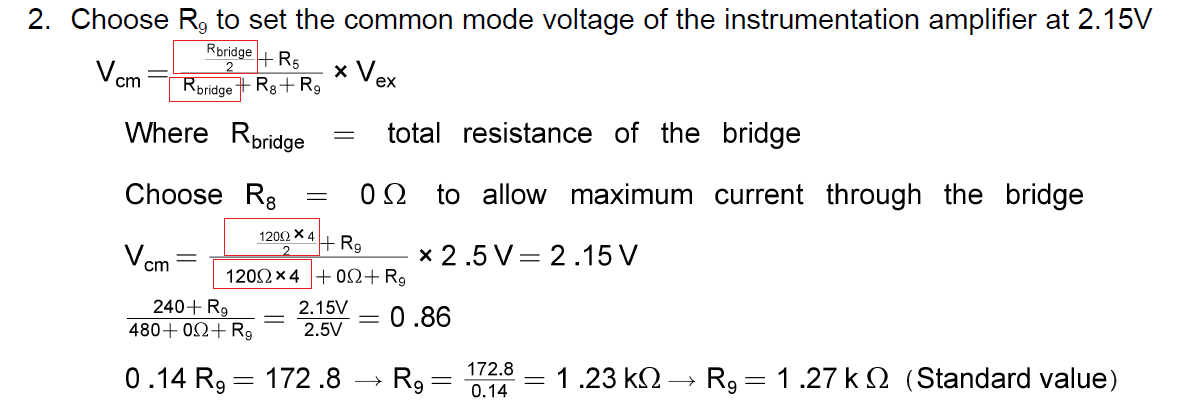If you have a related question, please click the "Ask a related question" button in the top right corner. The newly created question will be automatically linked to this question.

# TLV9002: Finding Vcm in Single-supply strain gauge bridge amplifier circuit

Part Number: TLV9002

Hi

Im having trouble when following the design steps (page 2 of the application note, or page 262 of  Analog Engineer’s Circuit Cookbook: Amplifiers Second Edition SLYY137 - 03/2019).

Where is this equation come from?

I know  Vcm = 0.5(Vex), but then how can I expand it to involve the resistances?

Fran

• Hi Fran,

A derivation of that equation is provided in "Single-Supply Strain Gauge in a Bridge Configuration Reference Design". Looking at the equation provided in the Analog Engineer's Circuit Cookbook, I believe there is a typo in the equation. R5 in the equation should be R9.

Lastly, I'm not sure what you mean by "how can I expand it to involve the resistances?" Rbridge is the equivalent resistance of the bridge and R8/9 are the resistors at the top and bottom of the bridge. By adding R9 or R8 to the circuit, you can shift the common mode voltage up or down, respectively. The common mode voltage of the bridge needs to be shifted up because it uses a 2.5V bridge excitation voltage and the op amps are supplied with a 5V supply. The document I reference earlier goes into more detail about this.

Thank you,

Tim Claycomb

• There's a typo in the formula. It must be R9 and not R5.

First, the current is calculated which flows through the Bridge:

I = Vex / (R8 + Rbridge + R9)

Then, Vcm is calculated:

Vcm = I * R9 + I / 2 * R7 = I * R9 + I / 2 * Rbridge

Vcm = (R9 / (R8 + Rbridge + R9) + 1/2 * Rbridge / (R8 + Rbridge + R9)) * Vex = (R9 + 1/2 * Rbridge) / (R8 + Rbridge + R9) * Vex

Kai

• Hi Kai

Thanks, the typo explained a lot of things now.

Fran

• Thanks Tim

the typo explains a lot.

Thanks for sending the other document. It also allowed  me to discovered the software tool to evaluate Vcm vs Vout on Opams.

Is that document part of another cookbook?

Also, can you please confirm there is another typo in further down in the analysis on the Analog Engineer's Circuit Cookbook.  It is implying that the Bridge equivalent resistor is 120 times 4. But I think that's incorrect, it should be the parallel sum of two 120 in series .• Hi Francisco,

this is another mistake :-)

Eliminate "x4".

For a common mode voltage of 2.15V I get R9 = 308.57Ohm.

Kai

• Hi Francisco,

I do not believe the reference design is part of another cookbook.

Yes, this appears to be another typo in the document that we will have to update. Thank you for bringing this to our attention.

Thank you,

Tim Claycomb

• OK thank you!

• You are welcome :-)

Kai